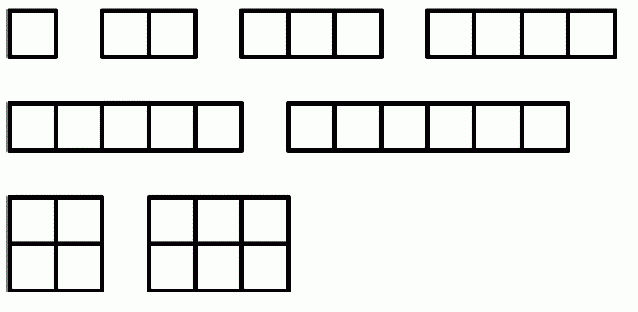Competitions

Rectangles

There is a collection of n squares with side 1. How many different rectangles can be formed using these squares?

Two rectangles are considered different if none of them can be rotated and moved to obtain the second one. During rectangle construction, its forbidden to deform the squares or to put any squares upon any other ones.

Input

One integer n (1n109).

Output

Print the number of different rectangles that can be formed using squares.Time limit 1 second
Memory limit 128 MiB
Input example #1
6
Output example #1
8
Author Azercell Cup, Round 1